# Pandas的crosstab函数

### 目录

• 简介

• 设置

• crosstab基础知识

• Pandas crosstab()与pivot_table()和groupby()的比较

• Pandas crosstab()的进一步定制

• Pandas crosstab()，多个组

### 设置

# 导入必要的库
import pandas as pd
import matplotlib.pyplot as plt
import seaborn as sns
import numpy as np

# 忽略警告
import warnings
warnings.filterwarnings('ignore')

# 启用多单元输出
from IPython.core.interactiveshell import InteractiveShell
InteractiveShell.ast_node_interactivity = 'all'

diamonds = sns.load_dataset('diamonds')
diamonds.head()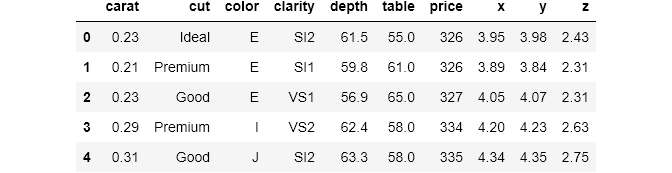### crosstab()基础知识

crosstab()总是返回一个数据帧，下面是一个例子。dataframe是diamonds中两个变量的交叉表：cut和color。交叉表表示取一个变量，将其组显示为index，取另一个变量，将其组显示为columns。

pd.crosstab(index=diamonds['cut'], columns=diamonds['color'])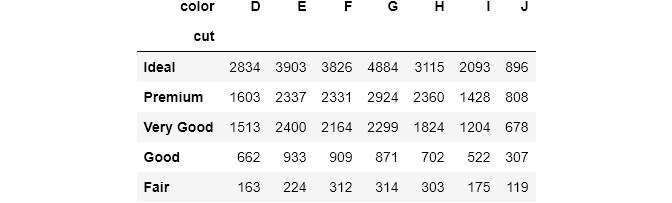pd.crosstab(index=diamonds['cut'],
columns=diamonds['color'],
values=diamonds['price'],
aggfunc=np.mean).round(0)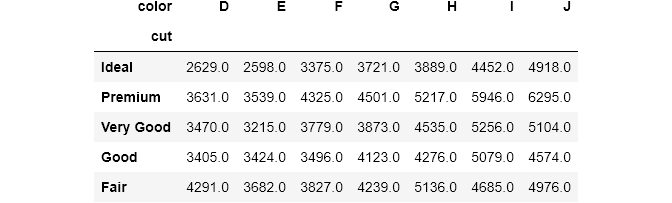cross = pd.crosstab(index=diamonds['cut'],
columns=diamonds['color'],
values=diamonds['price'],
aggfunc=np.mean).round(0)
sns.heatmap(cross, cmap='rocket_r', annot=True, fmt='g');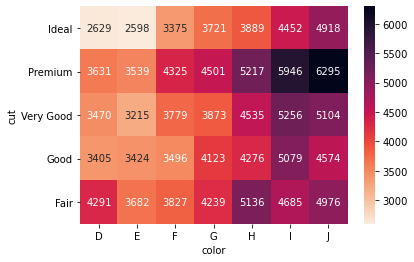seaborn可以自动将crosstab()表转换为热图。我将注释设置为True，并用颜色条显示热图。seaborn还为列和索引名添加了样式(fmt=’g’ 将数字显示为整数而不是科学计数)。

def plot_heatmap(cross_table, fmt='g'):
fig, ax = plt.subplots(figsize=(8, 5))
sns.heatmap(cross_table,
annot=True,
fmt=fmt,
cmap='rocket_r',
linewidths=.5,
ax=ax)
plt.show();

### Pandas crosstab()与pivot_table()和groupby()的比较

# 使用 groupby()
>>> diamonds.groupby(['cut', 'color'])['price'].mean().round(0)

cut        color
Ideal      D        2629.0
E        2598.0
F        3375.0
G        3721.0
H        3889.0
I        4452.0
J        4918.0
E        3539.0
F        4325.0
G        4501.0
H        5217.0
I        5946.0
J        6295.0
Very Good  D        3470.0
E        3215.0
F        3779.0
G        3873.0
H        4535.0
I        5256.0
J        5104.0
Good       D        3405.0
E        3424.0
F        3496.0
G        4123.0
H        4276.0
I        5079.0
J        4574.0
Fair       D        4291.0
E        3682.0
F        3827.0
G        4239.0
H        5136.0
I        4685.0
J        4976.0
Name: price, dtype: float64

# 使用 pivot_table()
diamonds.pivot_table(values='price',
index='cut',
columns='color',
aggfunc=np.mean).round(0)
# 使用 crosstab()
pd.crosstab(index=diamonds['cut'],
columns=diamonds['color'],
values=diamonds['price'],
aggfunc=np.mean).round(0)grouped = diamonds.groupby(['cut', 'color'])['price'].mean().round(0)
grouped.unstack()%%timeit
diamonds.pivot_table(values='price',
index='cut',
columns='color',
aggfunc=np.mean)
11.5 ms ± 483 µs per loop (mean ± std. dev. of 7 runs, 100 loops each)

%%timeit
pd.crosstab(index=diamonds['cut'],
columns=diamonds['color'],
values=diamonds['price'],
aggfunc=np.mean)
10.8 ms ± 344 µs per loop (mean ± std. dev. of 7 runs, 100 loops each)

%%timeit
diamonds.groupby(['cut', 'color'])['price'].mean().unstack()
4.13 ms ± 39.8 µs per loop (mean ± std. dev. of 7 runs, 100 loops each)

• 如果传递了all或True，则将规范化所有值。

• 如果传递index，将规范化每一行。

• 如果传递columns，将规范化每个列。

cross = pd.crosstab(index=diamonds['cut'],
columns=diamonds['color'],
normalize='all')
plot_heatmap(cross, fmt='.2%')# 证明所有值加起来约等于1
>>> pd.crosstab(diamonds['cut'],
diamonds['color'],
normalize='all').values.sum()

1.0000000000000002

cross = pd.crosstab(diamonds['cut'],
diamonds['color'],
normalize='index')
plot_heatmap(cross, fmt='.2%')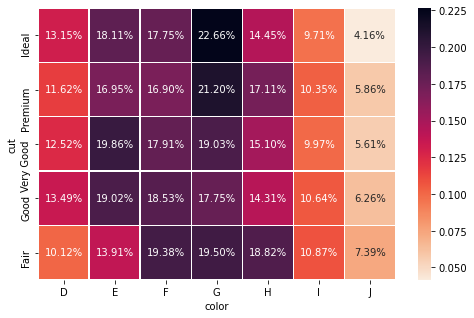cross = pd.crosstab(diamonds['cut'], diamonds['color'], normalize='columns')
plot_heatmap(cross, fmt='.2%')diamonds.pivot_table(index='color',
columns='cut',
fill_value=0)

pd.crosstab(diamonds['cut'], diamonds['color']).fillna(0)

### Pandas crosstab()的进一步定制

crosstab()的另外两个有用参数是margins和margins_name(两者都存在于pivot_table()中)。设置为True时，边界计算每行和每列的和。我们来看一个例子：

pd.crosstab(index=diamonds['cut'],
columns=diamonds['clarity'],
margins=True)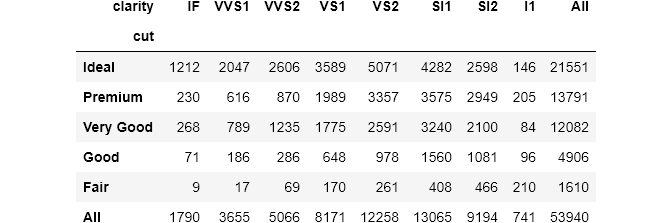pandas自动添加最后一行和最后一列，默认名称为All。margins_name可以控制名字：

pd.crosstab(index=diamonds['cut'],
columns=diamonds['clarity'],
margins=True,
margins_name='Total Number')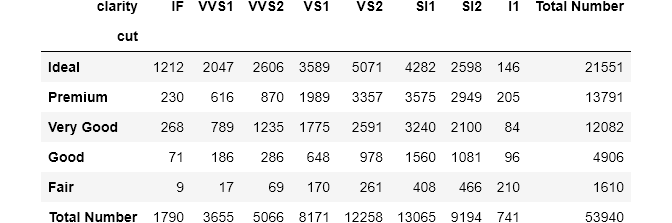pd.crosstab(index=diamonds['cut'],
columns=diamonds['clarity'],
margins=True,
margins_name='Total Percentage',
normalize=True)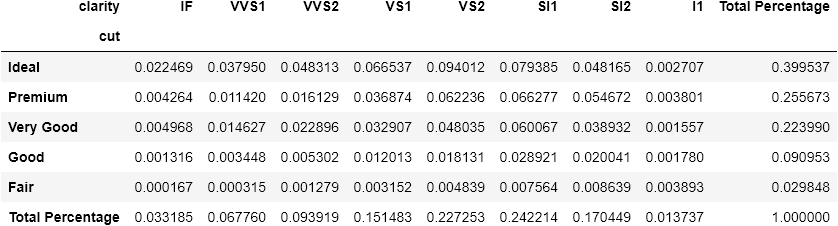### Pandas crosstab()，多组

pd.crosstab(index=[diamonds['cut'], diamonds['clarity']],
columns=diamonds['color'])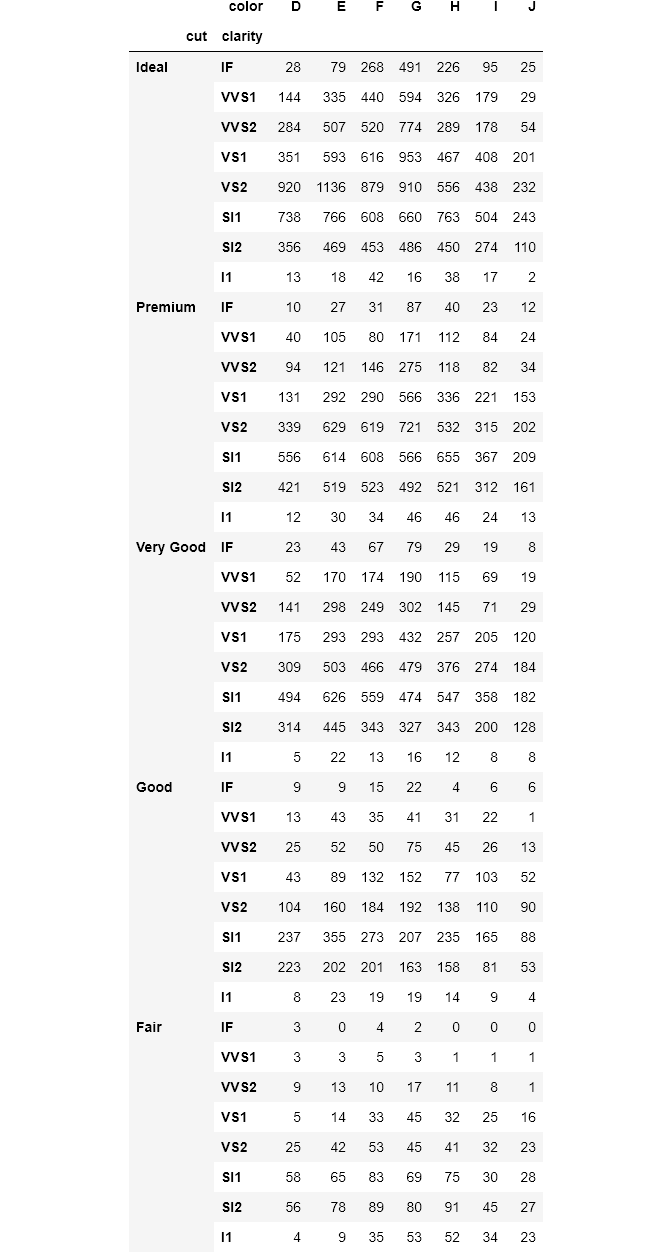pd.crosstab(index=[diamonds['cut'], diamonds['clarity']],
columns=diamonds['color'],
rownames=['Diamond Cut', 'Clarity']).head()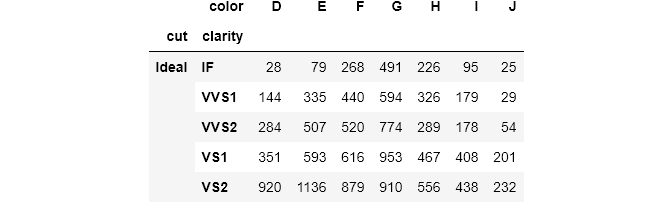http://panchuang.net/

sklearn机器学习中文官方文档：
http://sklearn123.com/

http://docs.panchuang.net/